# Basic principles of system modeling

Models are an essential tool for engineers. When developing a new product it is critical to asses its behavior in various situations. In most of the cases a full scale prototype of the new product is not available to be subject of testing.

Imagine that you need to design a new airplane or a new vehicle. But, before actually starting to build a prototype, you must be relatively sure that your design will meet certain criteria in terms of: cost, performance and durability. They only possible way to asses the criteria, before having a prototype, is by using a model.

A model can be anything, depending on the needs of the user. For example, in order to asses the aerodynamics of a body, a clay model of a vehicle at smaller scale is enough. For vibration analysis a body mass with dampers could be sufficient.

Models can be real (scale models) or virtual. Computers nowadays allow us to develop complex models, with multi-body, thermal, electrical or hydraulic components. The process to create a model is called modeling.Image: Radio controlled airplane model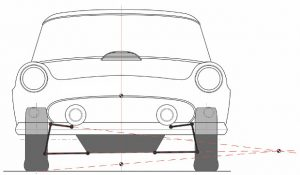Image: Vehicle suspension model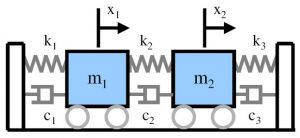Image: Vibration model with damping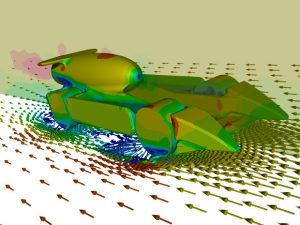Image: CFD (Computational Fluid Dynamics) simulation model

Everything around us is continuously changing. A system whose status changes within time is called a dynamic system. Most of the aspects of modern engineering are linked to dynamic systems, which has a significant impact in market competition and product development.

There is a ever-growing demand for better, faster products for the market. In the same time, the engineering products become more and more complex in terms of components and functionality. Because of all these aspects, dynamic systems are a particular interest for modeling.

In order to understand and predict dynamic behaviors of existing system, as well as to design new systems, engineers need to use computer-assisted modeling, simulation and analysis tools. In this context, modeling will be the process of creating a simplified representation of the features of a real dynamic system that are representative (relevant to their dynamic behavior).

A model is an abstraction of the real system, is a representation of reality. Through modeling we mimic the relevant features of the system being analyzed. A model can be represented in form of equations, diagrams or tables of data.

Dynamic systems are described by differential equations. The solution of the differential equation shows how the variables of the system depend on the independent variable (which is time).

For example, in order to study the vertical dynamics of a simple MacPherson strut suspension on the left front wheel of a rear-wheel drive vehicle, we can use a simple model of a mass with a spring and damper.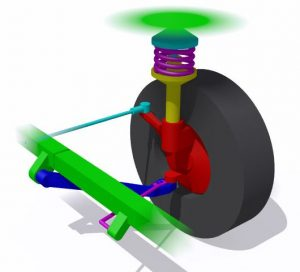Image: Simple MacPharson strut suspension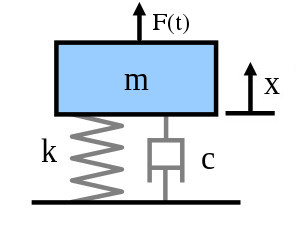Image: Forced mass spring damper model

To understand the behavior of the system in certain conditions, we need to describe it in mathematical terms. With this purpose in mind, for the suspension system model, we need to write down the governing differential equations:

$m \cdot \frac{d^2x}{dt^2} + c \cdot \frac{dx}{dt} + k \cdot x = F(t)$

where:

m [kg] – wheel mass
c [N·s/m] – viscous damping coefficient
k [N/m] – spring stiffness coefficient
x [m] – wheel displacement (position)
dx/dt [m/s] – wheel vertical speed
d2x/dt2 [m/s2] – wheel vertical acceleration
F(t) [N] – input force

Solving the differential equation we will be able to see the impact of the input force on the wheel movement for different suspension parameters (damping and stiffness)

Through modeling we have converted our suspension into a system, with input, output, states and parameters.

For the purpose of dynamic system analysis we will use mathematical models, described by differential equations. These models can describe mechanical, electrical, hydraulic or thermal systems. To summarize, a mathematical model is:

• a mathematical representation of the behavior of a real system, object or process
• a simplified, abstract, mathematical description related to a part of the real system, created with a specific purpose
• a set of equations (differential and/or algebraic) which describes the relationships between the system’s variables

For any questions, observations and queries regarding this article, use the comment form below.

Don’t forget to Like, Share and Subscribe!